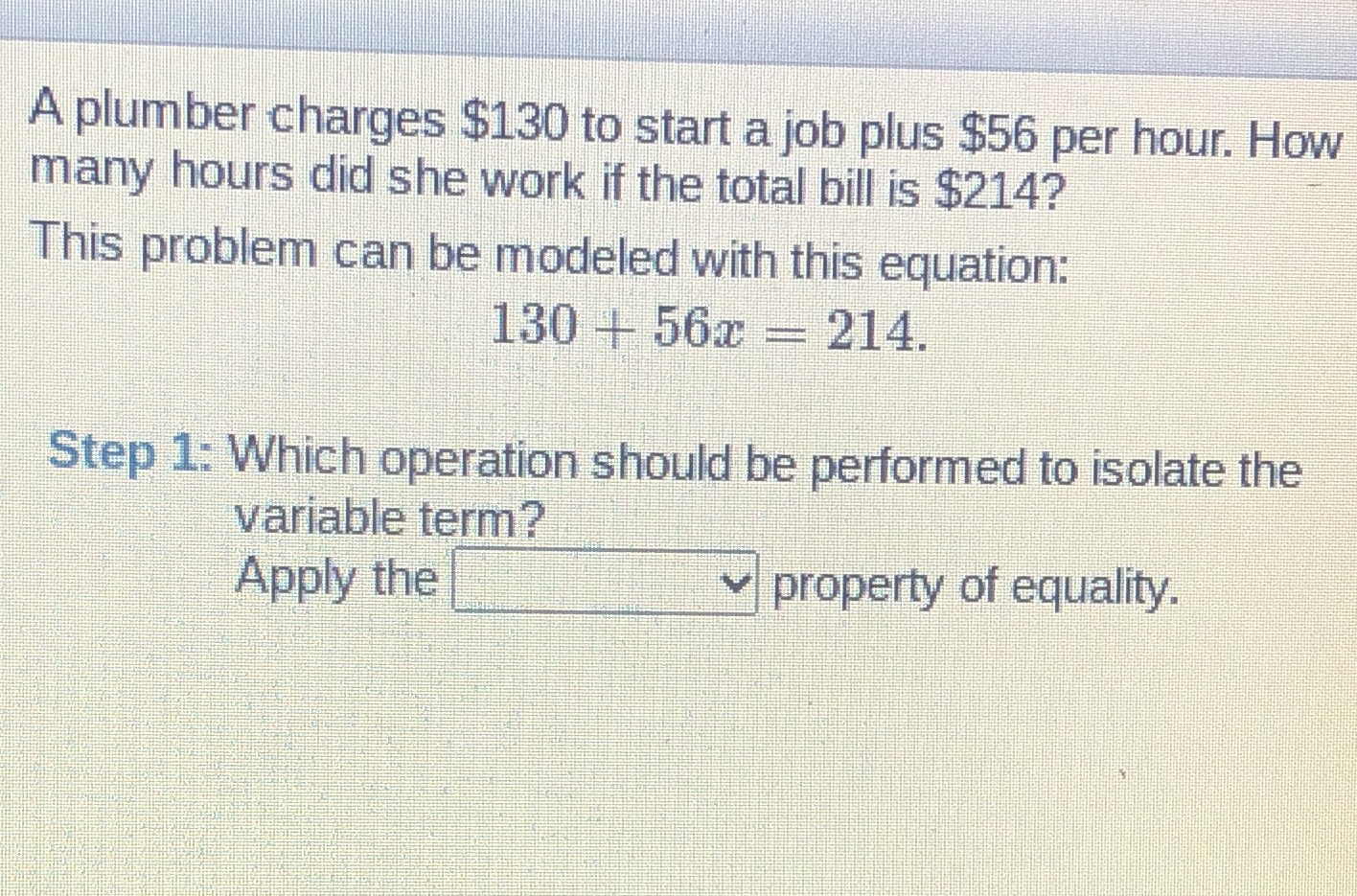### ¿Todavía tienes preguntas de matemáticas?

Pregunte a nuestros tutores expertos
Algebra
PreguntaA plumber charges $$\ 130$$ to start a job plus $$\ 56$$ per hour. How many hours did she work if the total bill is $$\ 214 ?$$ This problem can be modeled with this equation: Step 1: Which operation should be performed to isolate the variable term? Apply the $$\square \vee$$ property of equality. $$130 + 56 x = 214$$ .